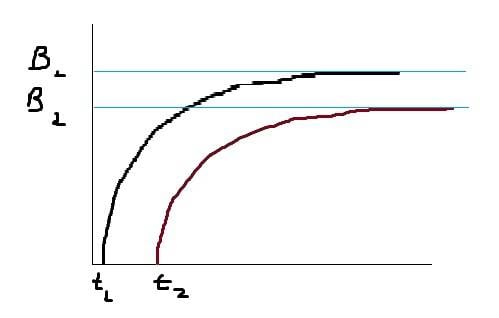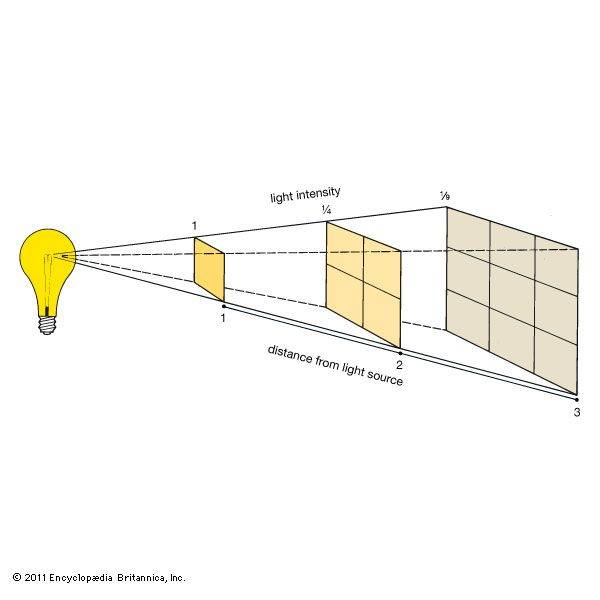# Electromagnetic Waves

• kent davidge

#### kent davidge

(I'm sorry for my poor English.) I draw a sketch showing the intensity of the magnetic field at two points p1 and p2, where the distance from the source (a wire carrying a current, for example) to p2 is greater than it's for p1. Is the shape of the curve correct? Is it correct that t2-t1/c is the distance traveled by the wave? Also, why is the final magnitude of B less in p2 than it's in p1 (what occurs in each point right after the wave pass)?Is the shape of the curve correct?

It is not. If t1 and t2 are distances, and the Y-axis is the intensity of the field, the curves should be start high on the left and get smaller as they go to the right.

Also, why is the final magnitude of B less in p2 than it's in p1 (what occurs in each point right after the wave pass)?

Because the intensity of EM waves falls of as 1/r2 as the wave spreads out into space as it travels. Note that this relationship is only true for waves that are expanding as a spherical wavefront or as a portion of a spherical wavefront. If this is not happening, such as if the wave is passing through an optical system, it does not follow this relationship.

Last edited:
Horizontal axis represent timeSo, is my reasoning correct?

Because the intensity of EM waves falls of as 1/r2 as the wave spreads out into space as it travels
But why does the wave intensity falls if there's no charge or anything "eating" its energy? Feynman says in his physics textbook that EM fields in the wavefront have the same intensity in every place where the wave travels.

But why does the wave intensity falls if there's no charge or anything "eating" its energy? Feynman says in his physics textbook that EM fields in the wavefront have the same intensity in every place where the wave travels.

I believe there are several fundamental reasons that the intensity drops off as the wave travels, but the easiest one to understand in my opinion is conservation of energy. If the wave kept the same intensity as it expanded outwards, energy conservation would be violated. The wave is able to perform the same amount of work everywhere along the wavefront, which requires energy. If the intensity doesn't drop, then the energy density doesn't decrease and the total amount of work able to be performed by the wave increases as the wave spreads out. Since the total amount of work done by the wave must equal the initial work required to create the wave, this would violate energy conservation.

•kent davidge
So, is my reasoning correct?

What reasoning? This part quoted below?

Is it correct that t2-t1/c is the distance traveled by the wave?

It is not. T2-T1 gives units in seconds. In order to get a final value with units of distance you'd need to multiply by c, not divide. For example:

T2-T1 = 0.1 s
0.1s * 300,000 m/s = 30,000 s*m/s = 30,000 m. (the two units of seconds cancel out since one is on the top of the fraction and one is on the bottom)

•kent davidge
But why does the wave intensity falls if there's no charge or anything "eating" its energy? Feynman says in his physics textbook that EM fields in the wavefront have the same intensity in every place where the wave travels.

because the same amount of power ( energy ) is getting spread out over a larger and larger area

as Drakkith said ...
Because the intensity of EM waves falls of as 1/r2 as the wave spreads out into space as it travels.

see this diag ... shows it very plainly ...Dave

•kent davidge
Drakkith
davenn

Thank you for your explanation. What about the amplitude of the wave? It falls with the distance (since the magnitude of the E & M fields drops)?

That's correct.

So why in most of the physics books a wave is described like y (x,t) = Acos(ωt + φ)
and they say the amplitude never changes.

So why in most of the physics books a wave is described like y (x,t) = Acos(ωt + φ)
and they say the amplitude never changes.
That's not the equation of a traveling wave, it's the equation of a standing plane wave. For a traveling wave you want something like ##A(x,t)=A_0\cos(kx-\omega{t}+\phi)##, which also has the property that the amplitude never changes - it is always ##A_0##.

The traveling wave described by that equation is a plane wave, meaning that its value at any moment is the same at all points in any plane perpendicular to the direction of travel. That's obviously not realistic ("all points" means out to infinity) but it is a perfectly good mathematical solution of the wave equation. Textbooks use this solution to the wave equation for several reasons, but for this discussion we only care about one: any physically realistic wave, including the spherically spreading one you and Drakkith have been discussing can be written as a sum of these infinite plane waves traveling in different directions.

When you write that spherical wave as such a sum of plane waves and then go to calculate the amplitude at each point, you'll find that the various plane waves contribute to the sum in such a way as to yield the inverse-square formula. Thus, the plane-wave formula in your textbook is just the starting point for a much more involved analysis of the more complicated spherical wave.

In practice, unless you need to dig deep into the general behavior of waves (mandatory for any serious study of physics, optional for the interested layman) you won't bother with these mathematical gyrations. You just look at the picture davenn posted, which provides a fine explanation of how the intensity drops with the square of the distance.

That's not the equation of a traveling wave, it's the equation of a standing plane wave. For a traveling wave you want something like ##A(x,t)=A_0\cos(kx-\omega{t}+\phi)##, which also has the property that the amplitude never changes - it is always ##A_0##.
Small correction the equation for standing plane wave should be ##A(x,t)=A_0\sin(kx)cos(\omega{t}+\phi)## where ##A_0## is plain constant.

Nugatory
I understood it now. Thank you.

https://www.physicsforums.com/members/delta.189563/